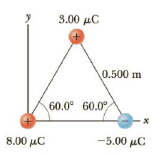Chapter 15, Problem 32P

Chapter
Section
Textbook Problem

Three charges are at the corners of an equilateral triangle, as shown in Figure P15.32. Calculate the electric field at a point midway between the two charges on the x-axis.Figure P15.32

To determine
The electric field at midpoint between the charges on the x axis.

Explanation

The vertical componentis directed downwards and due to the electric field of charge present at the top. The horizontal component is the sum of the fields due to the two charges on the x axis.

Formula to calculate the electric field due to 3.00μC is,

E1=keq1r12

• ke is the Coulomb constant.
• q1 is the top charge.
• r1 is the distance between the top charge and the point.

Formula to calculate the electric field due to 8.00μC is,

E2=keq2r22

• q2 is the charge at the origin.
• r2 is the distance between the charge at the origin and the point.

Formula to calculate the electric field due to 5.00μC is,

E3=keq3r32

• q3 is the negative charge.
• r2 is the distance between the charge at the origin and the point.

The horizontal component of electric field at the point is,

Ex=E2+E3

The vertical component of electric field at the point is,

Ey=E1

The net electric field at the point is,

Enet=Ex2+Ey2

Substitute for Ex and Ey in the above equation.

Enet=(keq2r22+keq3r32)+(keq1r12)2=ke(q2r22+q3r32)2+(q1r12)2

Substitute the values for the charges.

Enet=(keq2r22+keq3r32)+(keq1r12)2=(8

Still sussing out bartleby?

Check out a sample textbook solution.

See a sample solution

The Solution to Your Study Problems

Bartleby provides explanations to thousands of textbook problems written by our experts, many with advanced degrees!

Get Started